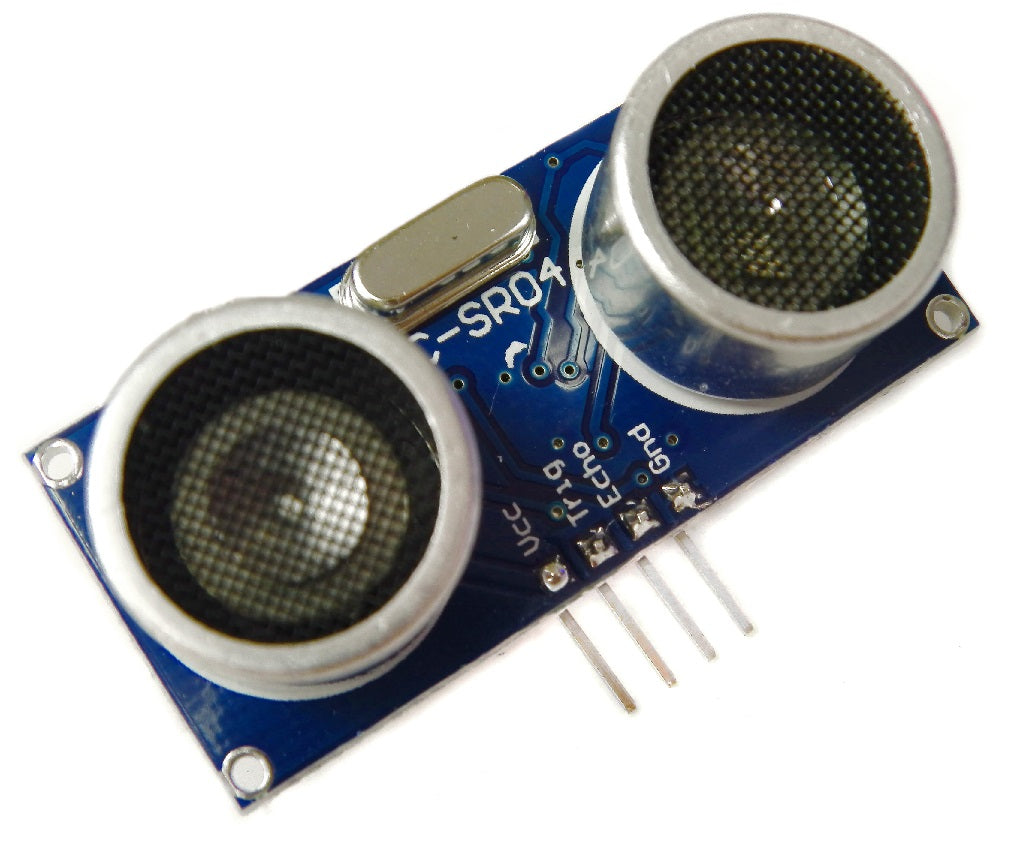# HC-SR04 Ultrasonic Range Sensor on the Raspberry Pi

In previous tutorials we've outlined temperature sensing, PIR motion controllers and buttons and switches, all of which can plug directly into the Raspberry Pi's GPIO ports. The HC-SR04 ultrasonic range finder is very simple to use, however the signal it outputs needs to be converted from 5V to 3.3V so as not to damage our Raspberry Pi! We'll introduce some Physics along with Electronics in this tutorial in order to explain each step!

What you'll need:

• HC-SR04
• 1kΩ Resistor
• 2kΩ Resistor
• Jumper Wires

Ultrasonic Distance Sensors

Sound consists of oscillating waves through a medium (such as air) with the pitch being determined by the closeness of those waves to each other, defined as the frequency. Only some of the sound spectrum (the range of sound wave frequencies) is audible to the human ear, defined as the “Acoustic” range. Very low frequency sound below Acoustic is defined as “Infrasound”, with high frequency sounds above, called “Ultrasound”. Ultrasonic sensors are designed to sense object proximity or range using ultrasound reflection, similar to radar, to calculate the time it takes to reflect ultrasound waves between the sensor and a solid object. Ultrasound is mainly used because it’s inaudible to the human ear and is relatively accurate within short distances. You could of course use Acoustic sound for this purpose, but you would have a noisy robot, beeping every few seconds. . . .

A basic ultrasonic sensor consists of one or more ultrasonic transmitters (basically speakers), a receiver, and a control circuit. The transmitters emit a high frequency ultrasonic sound, which bounce off any nearby solid objects. Some of that ultrasonic noise is reflected and detected by the receiver on the sensor. That return signal is then processed by the control circuit to calculate the time difference between the signal being transmitted and received. This time can subsequently be used, along with some clever math, to calculate the distance between the sensor and the reflecting object.The HC-SR04 Ultrasonic sensor we’ll be using in this tutorial for the Raspberry Pi has four pins: ground (GND), Echo Pulse Output (ECHO), Trigger Pulse Input (TRIG), and 5V Supply (Vcc). We power the module using Vcc, ground it using GND, and use our Raspberry Pi to send an input signal to TRIG, which triggers the sensor to send an ultrasonic pulse. The pulse waves bounce off any nearby objects and some are reflected back to the sensor. The sensor detects these return waves and measures the time between the trigger and returned pulse, and then sends a 5V signal on the ECHO pin.

ECHO will be “low” (0V) until the sensor is triggered when it receives the echo pulse. Once a return pulse has been located ECHO is set “high” (5V) for the duration of that pulse. Pulse duration is the full time between the sensor outputting an ultrasonic pulse, and the return pulse being detected by the sensor receiver. Our Python script must therefore measure the pulse duration and then calculate distance from this.

IMPORTANT. The sensor output signal (ECHO) on the HC-SR04 is rated at 5V. However, the input pin on the Raspberry Pi GPIO is rated at 3.3V. Sending a 5V signal into that unprotected 3.3V input port could damage your GPIO pins, which is something we want to avoid! We’ll need to use a small voltage divider circuit, consisting of two resistors, to lower the sensor output voltage to something our Raspberry Pi can handle.

Voltage Dividers

A voltage divider consists of two resistors (R1 and R2) in series connected to an input voltage (Vin), which needs to be reduced to our output voltage (Vout). In our circuit, Vin will be ECHO, which needs to be decreased from 5V to our Vout of 3.3V.The following circuit and simple equation can be applied to many applications where a voltage needs to be reduced. If you don’t want to learn the techy bit, just grab 1 x 1kΩ and 1 x 2kΩ resistor.Without getting too deep into the math side, we only actually need to calculate one resistor value, as it’s the dividing ratio that’s important. We know our input voltage (5V), and our required output voltage (3.3V), and we can use any combination of resistors to achieve the reduction. I happen to have a bunch of extra 1kΩ resistors, so I decided to use one of these in the circuit as R1.

Plugging our values in, this would be the following: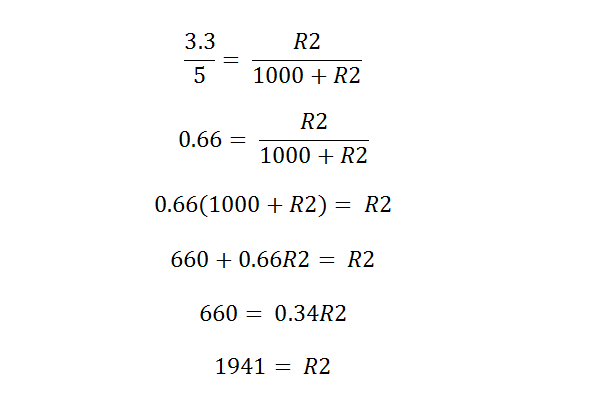So, we’ll use a 1kΩ for R1 and a 2kΩ resistor as R2!

Assemble the Circuit

We’ll be using four pins on the Raspberry Pi for this project: GPIO 5V [Pin 2]; Vcc (5V Power), GPIO GND [Pin 6]; GND (0V Ground), GPIO 23 [Pin 16]; TRIG (GPIO Output) and GPIO 24 [Pin 18]; ECHO (GPIO Input)1. Plug four of your male to female jumper wires into the pins on the HC-SR04 as follows: Red; Vcc, Blue; TRIG, Yellow; ECHO and Black; GND.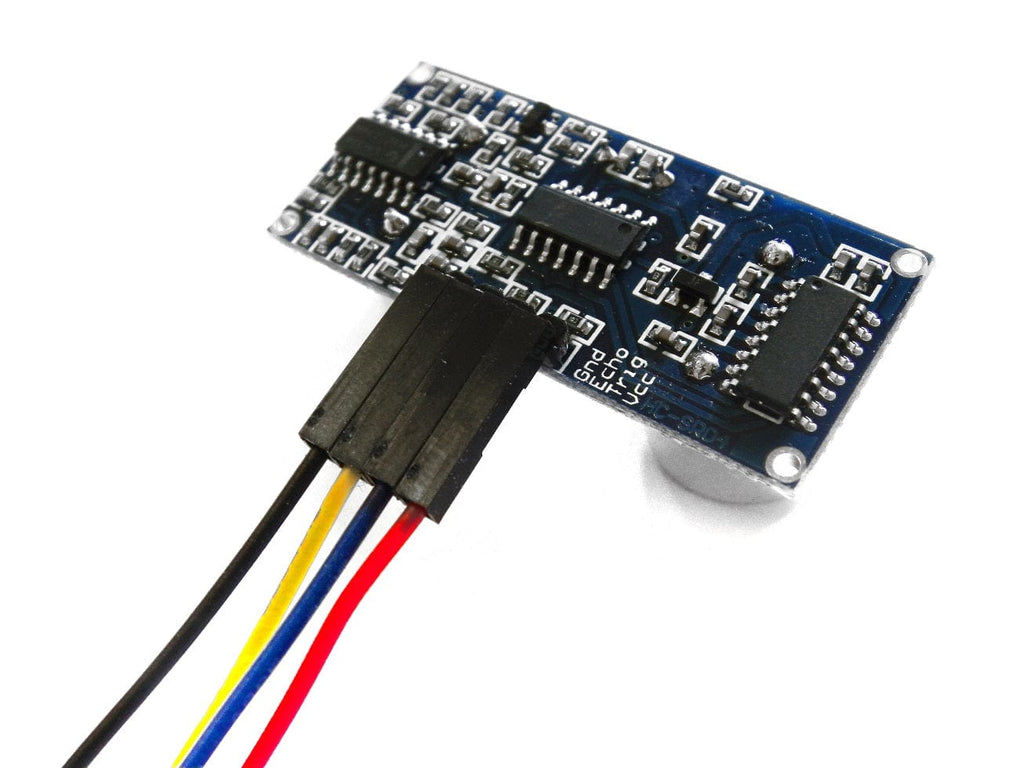2. Plug Vcc into the positive rail of your breadboard, and plug GND into your negative rail.

3. Plug GPIO 5V [Pin 2] into the positive rail, and GPIO GND [Pin 6] into the negative rail.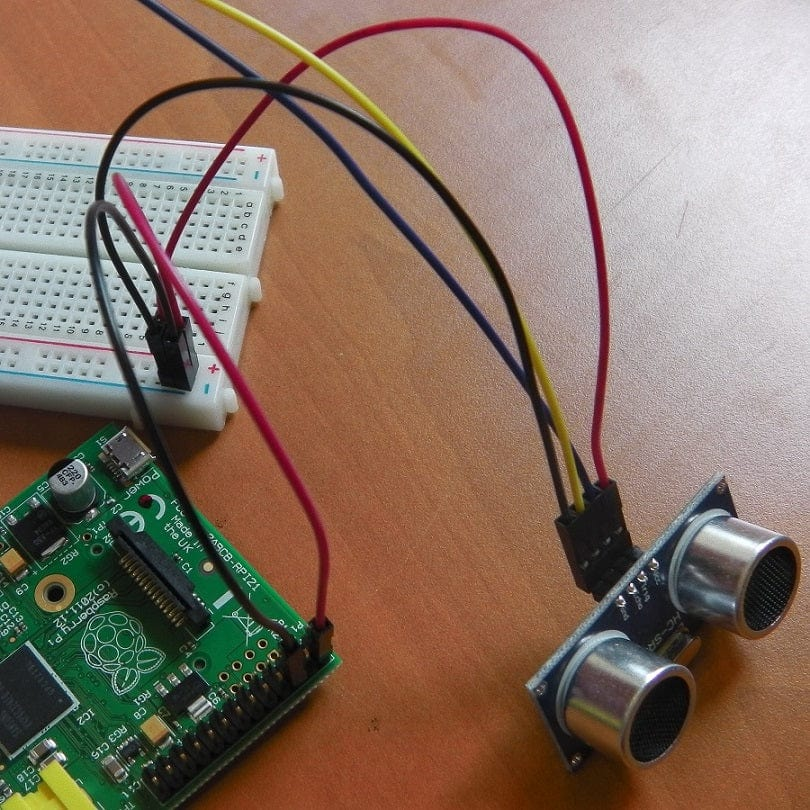4. Plug TRIG into a blank rail, and plug that rail into GPIO 23 [Pin 16]. (You can plug TRIG directly into GPIO 23 if you want). I personally just like to do everything on a breadboard!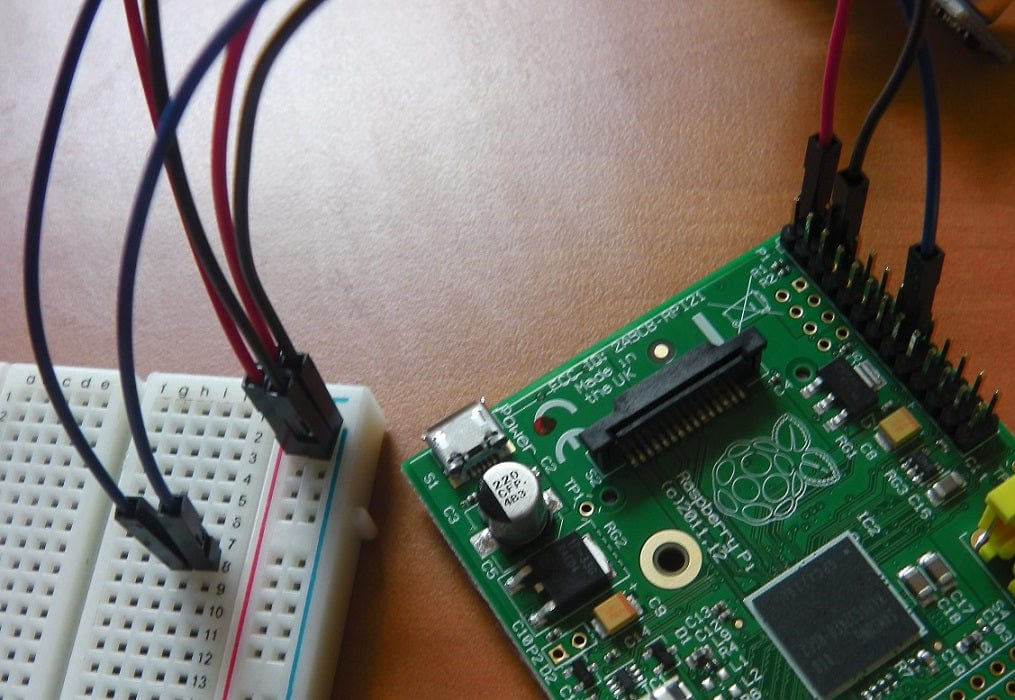5. Plug ECHO into a blank rail, link another blank rail using R1 (1kΩ resistor)

6. Link your R1 rail with the GND rail using R2 (2kΩ resistor). Leave a space between the two resistors.7. Add GPIO 24 [Pin 18] to the rail with your R1 (1kΩ resistor). This GPIO pin needs to sit between R1 and R2That's it! Our HC-SR04 sensor is connected to our Raspberry Pi!Sensing with Python

Now that we’ve hooked our Ultrasonic Sensor up to our Pi, we need to program a Python script to detect distance!

The Ultrasonic sensor output (ECHO) will always output low (0V) unless it’s been triggered in which case it will output 5V (3.3V with our voltage divider!). We therefore need to set one GPIO pin as an output, to trigger the sensor, and one as an input to detect the ECHO voltage change.

First, import the Python GPIO library, import our time library (so we make our Pi wait between steps) and set our GPIO pin numbering.

import RPi.GPIO as GPIO

import time

GPIO.setmode(GPIO.BCM)

Next, we need to name our input and output pins, so that we can refer to it later in our Python code. We’ll name our output pin (which triggers the sensor) GPIO 23 [Pin 16] as TRIG, and our input pin (which reads the return signal from the sensor) GPIO 24 [Pin 18] as ECHO.

TRIG = 23

ECHO = 24

We’ll then print a message to let the user know that distance measurement is in progress. . . .

print "Distance Measurement In Progress"

Next, set your two GPIO ports as either inputs or outputs as defined previously.

GPIO.setup(TRIG,GPIO.OUT)

GPIO.setup(ECHO,GPIO.IN)

Then, ensure that the Trigger pin is set low, and give the sensor a second to settle.

GPIO.output(TRIG, False)

print "Waiting For Sensor To Settle"

time.sleep(2)

The HC-SR04 sensor requires a short 10uS pulse to trigger the module, which will cause the sensor to start the ranging program (8 ultrasound bursts at 40 kHz) in order to obtain an echo response. So, to create our trigger pulse, we set out trigger pin high for 10uS then set it low again.

GPIO.output(TRIG, True)

time.sleep(0.00001)

GPIO.output(TRIG, False)

Now that we’ve sent our pulse signal we need to listen to our input pin, which is connected to ECHO. The sensor sets ECHO to high for the amount of time it takes for the pulse to go and come back, so our code therefore needs to measure the amount of time that the ECHO pin stays high. We use the “while” string to ensure that each signal timestamp is recorded in the correct order.

The time.time() function will record the latest timestamp for a given condition. For example, if a pin goes from low to high, and we’re recording the low condition using the time.time() function, the recorded timestamp will be the latest time at which that pin was low.

Our first step must therefore be to record the last low timestamp for ECHO (pulse_start) e.g. just before the return signal is received and the pin goes high.

while GPIO.input(ECHO)==0:

pulse_start = time.time()

Once a signal is received, the value changes from low (0) to high (1), and the signal will remain high for the duration of the echo pulse. We therefore also need the last high timestamp for ECHO (pulse_end).

while GPIO.input(ECHO)==1:

pulse_end = time.time()

We can now calculate the difference between the two recorded timestamps, and hence the duration of pulse (pulse_duration).

pulse_duration = pulse_end - pulse_start

With the time it takes for the signal to travel to an object and back again, we can calculate the distance using the following formula.The speed of sound is variable, depending on what medium it’s travelling through, in addition to the temperature of that medium. However, some clever physicists have calculated the speed of sound at sea level so we’ll take our baseline as the 343m/s. If you’re trying to measure distance through water, this is where you’re falling down – make sure you’re using the right speed of sound!

We also need to divide our time by two because what we’ve calculated above is actually the time it takes for the ultrasonic pulse to travel the distance to the object and back again. We simply want the distance to the object! We can simplify the calculation to be completed in our Python script as follows:We can plug this calculation into our Python script:

distance = pulse_duration x 17150

Now we need to round our distance to 2 decimal places (for neatness!)

distance = round(distance, 2)

Then, we print the distance. The below command will print the word “Distance:” followed by the distance variable, followed by the unit “cm”

print "Distance:",distance,"cm"

Finally, we clean our GPIO pins to ensure that all inputs/outputs are reset

GPIO.cleanup()Save your python script, I called ours "range_sensor.py", and run it using the following command. Running a root (sudo), is important with this script:The sensor will settle for a few seconds, and then record your distance!

Sources

Thanks for the following sources for information on this tutorial:

Raspberry Pi Spy - Part 1

Raspberry Pi Spy - Part 2

### 1 commentPeter

How do you improve the accuracy of the SR04 ? I have readings that sometimes jump erratically.

How do you improve the accuracy of the SR04 ? I have readings that sometimes jump erratically.